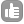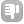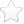1Hello, I'm trying to use an NMR spectrum to define/calculate a "degree of acrylation" for a PLA-PEG-PLA block copolymer that is di-acrylated. The macromer or (macro-monomer) is symmetrical in nature and looks like this: (Acr)p-(PLA)x-(PEG)m-(PLA)x-(Acr)p (-CH2-CH-CO-)p-(O-CH[CH3]-CO-)x-(CH2-CH2-O-)m-(O-CH[CH3]-CO-)x-(-CH2-CH-CO)p For the PEG block in the middle, m is large (I have 4 different samples with pre-defined PEG MWs of 2k, 4k, 8k, and 12k g/mol). For the PLA blocks on either side of the PEG block, I have been able to calculate the average values of x for each sample, using the known value of m for the PEG blocks and the NMR-integrated ratio of PLA CH peaks (at 5.2 ppm) to the main-chain PEG CH2 peaks (at 3.6 ppm). So the formula set-up for that looks like this: m = (PEG MW) / (EG unit MW) 2x / m = PLA CH peak / PEG CH2 peak x is typically small (x = 2 - 6 LA units per chain end). In order to calculate the average value of p and thereby define a "degree of acrylation," I should be able to use a similar process. Presumably, by integrating three acrylate group peaks (~ 6 ppm) compared to a shifted PEG peak that represents the PEG CH2 groups directly next to the PLA blocks (~ 4.3 ppm). However, I have a few questions: 1) Why do the acrylate groups gives 3 peaks even though they only contain two types of hydrogens (CH2 and CH)? 2) Is it correct to assume that the combined integration of the acrylate peaks proportionally represent (2H+1H) x 2 end groups = 6H's? 3) Would it be correct, assuming there are two PEG CH2 groups next to PLA blocks, per chain, that the peak at 4.3 proportionally represents 4 H's? 4) Since the desire in synthesizing these macromers is to obtain p = 1 (an average of one acrylate group per chain end) then does it make sense if I just define the "degree of acrylation" as p/1? 5) To calculate p using the NMR peaks: This is supposed to be possible by integrating the three acrylate peaks (~ 6 ppm) compared to a shifted PEG peak that represents the PEG CH2 groups directly next to the PLA blocks (~ 4.3 ppm). Since there are 2 such CH2 groups per chain as well as 2 acrylate groups per chain, would the ratio of the two peaks directly give me the value of p? For instance, if t refers to the number of CH2 groups per chain located directly next to PLA blocks, then using a formula similar to that above: 2p / t = Acr group peaks / shifted PEG CH2 group peak Since we know t = 2, then I can directly calculate p? asked Mar 09 '10 at 19:28juxtabo 11 thanks for re-organizing your post, much better this way! - Evgeny Fadeev (Mar 16 '10 at 14:58) one last thing, in polyacrylate - isn't there free -COOH "hanging in the air" sorry, I was confused about the structure at first. - Evgeny Fadeev (Mar 16 '10 at 15:00)1Question 1) The acrylate monomer has 3 peaks as the =CH2 generates 2 peaks, the 2 olefinic Hs are not equivalent, and the -CH one peak. Question 2) yes Question 3) yes Obs -- The acrylate block of the co-polymer has probably signals at ~ 2-2.5 ppm. Wouldn't that be of interest to calculate the ratio of the blocks acrylate/PEG? Knowing this ratio and the initial quantities of the co-monomers, it would be possible to calculate how many units of acrylate co-monomers are present in the polymer. The degree of acrylation would be calculated by the co-monomer directly incorporated in the co-polymer. answered Mar 10 '10 at 12:370Looks like the repeating unit is -(Acr)p-(PLA)x-(PEG)m-(PLA)x-. Yes, the integral ratios should work and it should be possible to determine for example `

` or `

`. After all the peaks are assigned :). How broad are the lines? Is it possible that you see three peaks because there should be a multiplet? Also, is it possible that certain conformation forces the protons in CH2 group become non-equivalent? Finally I thought that in the cases where p=1 and p!=1 chemical shifts of acrylic residue will probably be different, no? For the experiment to give correct integral ratios it's important to make sure that none of the resonances are even partially saturated. That can be achieved by using small flip angle for the pulse - maybe 20 degrees and allow sufficient relaxation (5*T1 is a good choice). Figure 4.3 on p. 113 of Timothy Claridge "High Resolution NMR Techniques in Organic Chemistry" had a good illustration of that. Being careful with the choice of recycling delay and pulse width will be especially important if spins of nuclei "whose" peaks the need to be integrated have substantially different T1 relaxation times, e.g. methyl vs methyne. If the lines are wide and overlapping, line fitting may be required (for example in Origin or Matlab) to extract the integrals. Also - with lorentzian lineshapes integral reset points must be quite far from the peak because of the wide tails of the Lorentzian. answered Mar 10 '10 at 13:29Evgeny Fadeev 577●1